# Soup from canteen

For how many people is 90 liters of soup enough if we assume 3/8 liter of soup per person in the canteen?

Result

n =  240

#### Solution:Leave us a comment of example and its solution (i.e. if it is still somewhat unclear...):

Showing 0 comments:Be the first to comment!#### To solve this example are needed these knowledge from mathematics:

Need help calculate sum, simplify or multiply fractions? Try our fraction calculator. Tip: Our volume units converter will help you with converion of volume units.

## Next similar examples:

1. Percent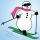From 99 children who participated in the ski course were 23 excellent skiers, 13 good and 20 average and the rest were beginners. Calculate this data in percentages.
2. Milk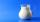At the kindergarten, every child got 1/5 liter of milk in the morning and another 1/8 liter of milk in the afternoon. How many liters were consumed per day for 20 children?
3. Vehicle tankA vehicle tank was 3/5 full of petrol. When 21 liters of fuel was added it was 5/6 full. How many liters of petrol can the tank hold?
4. Container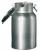How many three-quarters liters jar fill 30 liters container?
5. Cleaning windowsCleaning company has to wash all the windows of the school. The first day washes one-sixth of the windows of the school, the next day three more windows than the first day and the remaining 18 windows washes on the third day. Calculate how many windows ha
6. Lemonade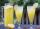How many 1/4 cup servings are in 2 and 1/3 cups of lemonade?
7. Sewing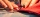Beth's mother can sew 235 pairs of short pants in 6 days while Lourdes can sew 187 pairs in 8 days. How many more pairs of short pants can Beth's mother sew?
8. Percents - easyHow many percent is 432 out of 434?
9. Base, percents, valueBase is 344084 which is 100 %. How many percent is 384177?
10. Percent calculationCalculate 61% if 85% is 38.
11. InqualitySolve inequality: 3x + 6 > 14
12. Typing course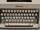Before taking a typing course, Terrence could type 39 words per minute. By the end of the course, he was able to type 68 words per minute. Find the percent increase.
13. Equation with xSolve the following equation: 2x- (8x + 1) - (x + 2) / 5 = 9
14. FractionsThree-quarters of an unknown number are 4/5. What is 5/6 of this unknown number?
15. UN 1If we add to an unknown number his quarter, we get 210. Identify unknown number.
16. Equation with fractionsSolve equation: ? It is equation with fractions.
17. Simple equation 6Solve equation with one variable: X/2+X/3+X/4=X+4